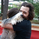479浏览
479
While trying to figure out how to make the STDEV function use an exponential moving average instead of simple moving average , I discovered the builtin function doesn't really use either.

Check it out, it's amazing how different the two-pass algorithm is from the builtin!

Eventually I reverse-engineered and discovered that STDEV uses the Naiive algorithm and doesn't apply "Bessel's Correction". K can be 0, it doesn't seem to change the data although having it included should make it a little more precise.

https://en.wikipedia.org/wiki/Algorithms...My conclusion is that Pine uses a single-pass algorithm which is known to have issues due to loss of precision when subtracting large numbers (on line 32). The way it accumulates the Ex and Ex2 numbers could lead to some error as well, but I don't think that is very significant in this case. ( You can check out my other study at to see how the 'cum' function has increasing error over time, but that is over a much larger set of numbers. )

The aqua and green lines here are actually more accurate than the builtin because they are doing the simple two-pass algorithm and so are working with much smaller numbers.

The document that I linked before ( http://cpsc.yale.edu/sites/default/files/files/tr222.pdf ) actually discusses this on page 1 and explicitly says, "Unfortunately, although is mathematically equivalent to , numerically it can be disastrous. The quantities and may be very large in practice, and will generally be computed with some rounding error. If the variance is small, these numbers should cancel out almost completely in the subtraction of . Many (or all) of the correctly compute digits will cancel, leaving a computed S with a possibly unacceptable relative error."sclark39
Reposting the quote since this stripped out my square brackets:

"Unfortunately, although (one-pass) is mathematically equivalent to (two-pass), numerically it can be disastrous. The quantities (Ex) and (Ex2) may be very large in practice, and will generally be computed with some rounding error. If the variance is small, these numbers should cancel out almost completely in the subtraction of (one-pass). Many (or all) of the correctly compute digits will cancel, leaving a computed S with a possibly unacceptable relative error."The question now is... which of these is actually more accurate? I have a suspicion the builtin one has a lot of precision error due to subtracting such large numbers.The explanation for the differences between these algorithms is explained in this document: http://cpsc.yale.edu/sites/default/files/files/tr222.pdf

Bonus: You can actually apply Bessel's Correction to the builtin function by doing:
stdev_w_bessel( src, len ) => sqrt( variance( src, len ) * len / ( len - 1 ) )